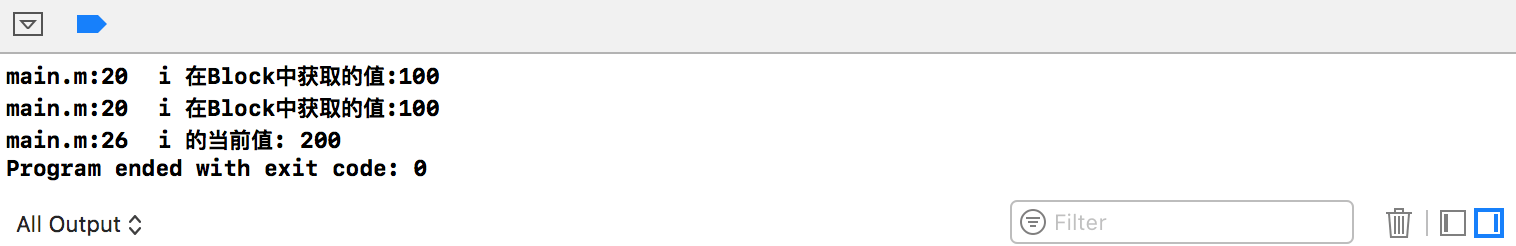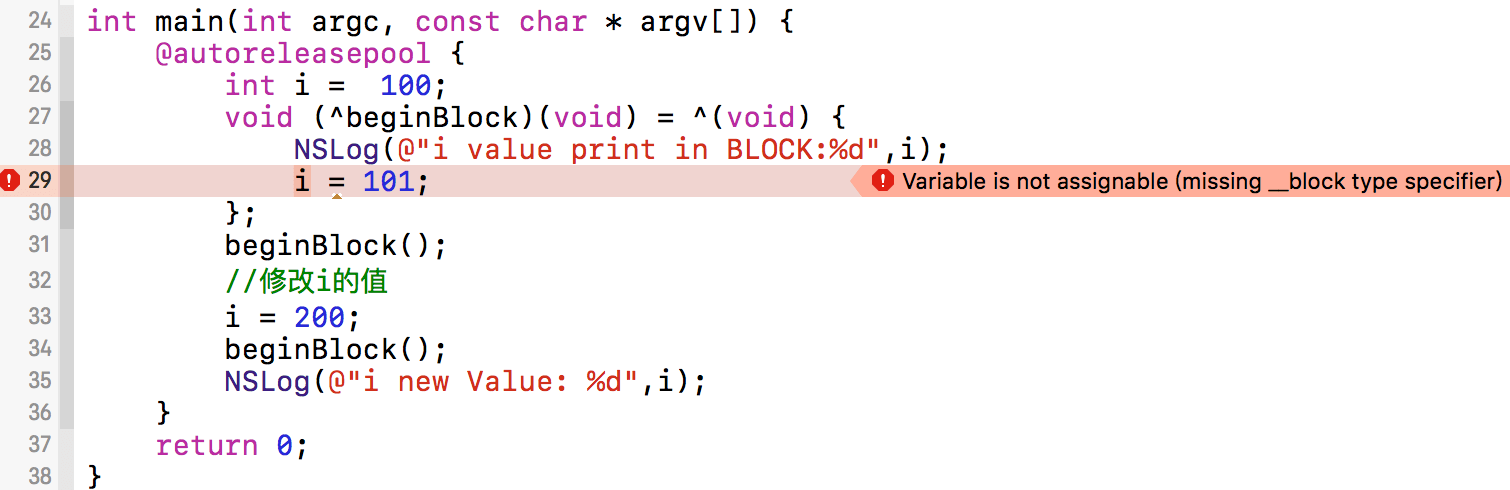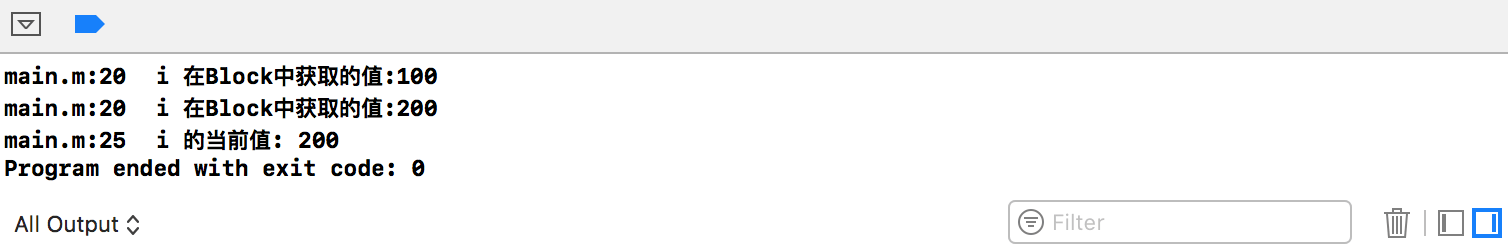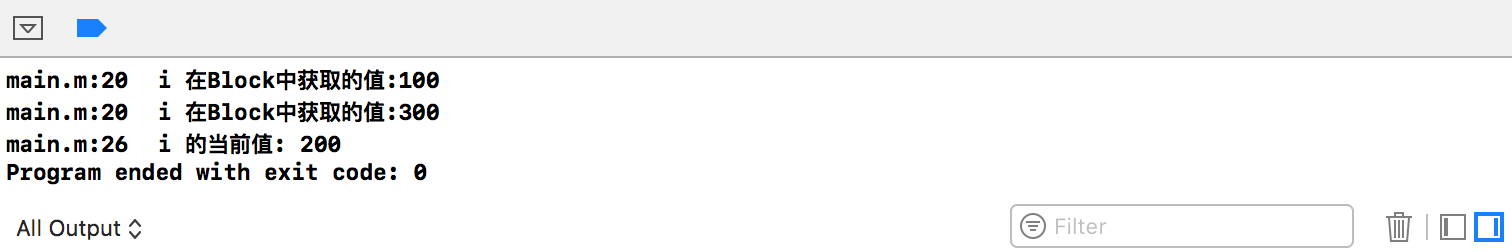# 1、访问Block之外的变量

``````int main(int argc, const char * argv[]) {
@autoreleasepool {
int i =  100;
void (^beginBlock)(void) = ^(void) {
NSLog(@"i 在Block中获取的值:%d",i);
};
beginBlock();
//修改i的值
i = 200;
beginBlock();
NSLog(@"i 的当前值: %d",i);
}
return 0;
}
``````# 2、修改Block之外的变量

``````int main(int argc, const char * argv[]) {
@autoreleasepool {
__block int i =  100;
void (^withBlockWord)(void) = ^(void) {
NSLog(@"i 在Block中获取的值:%d",i);
};
withBlockWord();
i = 200;
withBlockWord();
NSLog(@"i 的当前值: %d",i);
}
return 0;
}
````````````int main(int argc, const char * argv[]) {
@autoreleasepool {
__block int i =  100;
void (^withBlockWord)(void) = ^(void) {
NSLog(@"i 在Block中获取的值:%d",i);
i = 200;
};
withBlockWord();
i = 300;
withBlockWord();
NSLog(@"i 的当前值: %d",i);
}
return 0;
}
``````# 示例代码

https://github.com/99ios/5.1.3